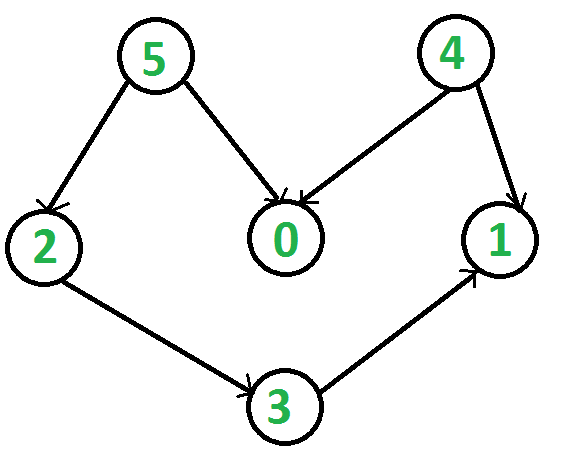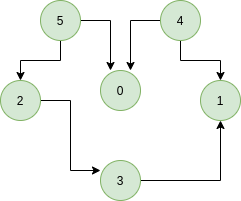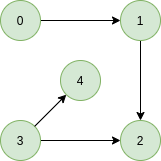Skip to content
Related Articles
Kahn’s algorithm for Topological Sorting
• Difficulty Level : Medium
• Last Updated : 31 May, 2020

Topological sorting for Directed Acyclic Graph (DAG) is a linear ordering of vertices such that for every directed edge uv, vertex u comes before v in the ordering. Topological Sorting for a graph is not possible if the graph is not a DAG.

For example, a topological sorting of the following graph is “5 4 2 3 1 0?. There can be more than one topological sorting for a graph. For example, another topological sorting of the following graph is “4 5 2 0 3 1″. The first vertex in topological sorting is always a vertex with in-degree as 0 (a vertex with no in-coming edges).Let’s look at few examples with proper explanation,
Example:

Input:Output: 5 4 2 3 1 0
Explanation: The topological sorting of a DAG is done in a order such that for every directed edge uv, vertex u comes before v in the ordering. 5 has no incoming edge. 4 has no incoming edge, 2 and 0 have incoming edge from 4 and 5 and 1 is placed at last.

Input:Output: 0 3 4 1 2
Explanation: 0 and 3 have no incoming edge, 4 and 1 has incoming edge from 0 and 3. 2 is placed at last.

## Recommended: Please solve it on “PRACTICE” first, before moving on to the solution.

A DFS based solution to find a topological sort has already been discussed.

Solution: In this article we will see another way to find the linear ordering of vertices in a directed acyclic graph (DAG). The approach is based on the below fact:

A DAG G has at least one vertex with in-degree 0 and one vertex with out-degree 0.
Proof: There’s a simple proof to the above fact is that a DAG does not contain a cycle which means that all paths will be of finite length. Now let S be the longest path from u(source) to v(destination). Since S is the longest path there can be no incoming edge to u and no outgoing edge from v, if this situation had occurred then S would not have been the longest path
=> indegree(u) = 0 and outdegree(v) = 0

Algorithm: Steps involved in finding the topological ordering of a DAG:
Step-1: Compute in-degree (number of incoming edges) for each of the vertex present in the DAG and initialize the count of visited nodes as 0.

Step-2: Pick all the vertices with in-degree as 0 and add them into a queue (Enqueue operation)

Step-3: Remove a vertex from the queue (Dequeue operation) and then.

1. Increment count of visited nodes by 1.
2. Decrease in-degree by 1 for all its neighboring nodes.
3. If in-degree of a neighboring nodes is reduced to zero, then add it to the queue.

Step 5: Repeat Step 3 until the queue is empty.

Step 5: If count of visited nodes is not equal to the number of nodes in the graph then the topological sort is not possible for the given graph.

How to find in-degree of each node?
There are 2 ways to calculate in-degree of every vertex:

1. Take an in-degree array which will keep track of
Traverse the array of edges and simply increase the counter of the destination node by 1.
```for each node in Nodes
indegree[node] = 0;
for each edge(src, dest) in Edges
indegree[dest]++```

Time Complexity: O(V+E)

2. Traverse the list for every node and then increment the in-degree of all the nodes connected to it by 1.
```    for each node in Nodes
If (list[node].size()!=0) then
for each dest in list
indegree[dest]++;```

Time Complexity: The outer for loop will be executed V number of times and the inner for loop will be executed E number of times, Thus overall time complexity is O(V+E).

The overall time complexity of the algorithm is O(V+E)

Below is C++ implementation of above algorithm. The implementation uses method 2 discussed above for finding indegrees.

## C++

 `// A C++ program to print topological``// sorting of a graph using indegrees.``#include ``using` `namespace` `std;`` ` `// Class to represent a graph``class` `Graph {``    ``// No. of vertices'``    ``int` `V;`` ` `    ``// Pointer to an array containing``    ``// adjacency listsList``    ``list<``int``>* adj;`` ` `public``:``    ``// Constructor``    ``Graph(``int` `V);`` ` `    ``// Function to add an edge to graph``    ``void` `addEdge(``int` `u, ``int` `v);`` ` `    ``// prints a Topological Sort of``    ``// the complete graph``    ``void` `topologicalSort();``};`` ` `Graph::Graph(``int` `V)``{``    ``this``->V = V;``    ``adj = ``new` `list<``int``>[V];``}`` ` `void` `Graph::addEdge(``int` `u, ``int` `v)``{``    ``adj[u].push_back(v);``}`` ` `// The function to do``// Topological Sort.``void` `Graph::topologicalSort()``{``    ``// Create a vector to store``    ``// indegrees of all``    ``// vertices. Initialize all``    ``// indegrees as 0.``    ``vector<``int``> in_degree(V, 0);`` ` `    ``// Traverse adjacency lists``    ``// to fill indegrees of``    ``// vertices.  This step``    ``// takes O(V+E) time``    ``for` `(``int` `u = 0; u < V; u++) {``        ``list<``int``>::iterator itr;``        ``for` `(itr = adj[u].begin();``             ``itr != adj[u].end(); itr++)``            ``in_degree[*itr]++;``    ``}`` ` `    ``// Create an queue and enqueue``    ``// all vertices with indegree 0``    ``queue<``int``> q;``    ``for` `(``int` `i = 0; i < V; i++)``        ``if` `(in_degree[i] == 0)``            ``q.push(i);`` ` `    ``// Initialize count of visited vertices``    ``int` `cnt = 0;`` ` `    ``// Create a vector to store``    ``// result (A topological``    ``// ordering of the vertices)``    ``vector<``int``> top_order;`` ` `    ``// One by one dequeue vertices``    ``// from queue and enqueue``    ``// adjacents if indegree of``    ``// adjacent becomes 0``    ``while` `(!q.empty()) {``        ``// Extract front of queue``        ``// (or perform dequeue)``        ``// and add it to topological order``        ``int` `u = q.front();``        ``q.pop();``        ``top_order.push_back(u);`` ` `        ``// Iterate through all its``        ``// neighbouring nodes``        ``// of dequeued node u and``        ``// decrease their in-degree``        ``// by 1``        ``list<``int``>::iterator itr;``        ``for` `(itr = adj[u].begin();``             ``itr != adj[u].end(); itr++)`` ` `            ``// If in-degree becomes zero,``            ``// add it to queue``            ``if` `(--in_degree[*itr] == 0)``                ``q.push(*itr);`` ` `        ``cnt++;``    ``}`` ` `    ``// Check if there was a cycle``    ``if` `(cnt != V) {``        ``cout << ``"There exists a cycle in the graph\n"``;``        ``return``;``    ``}`` ` `    ``// Print topological order``    ``for` `(``int` `i = 0; i < top_order.size(); i++)``        ``cout << top_order[i] << ``" "``;``    ``cout << endl;``}`` ` `// Driver program to test above functions``int` `main()``{``    ``// Create a graph given in the``    ``// above diagram``    ``Graph g(6);``    ``g.addEdge(5, 2);``    ``g.addEdge(5, 0);``    ``g.addEdge(4, 0);``    ``g.addEdge(4, 1);``    ``g.addEdge(2, 3);``    ``g.addEdge(3, 1);`` ` `    ``cout << ``"Following is a Topological Sort of\n"``;``    ``g.topologicalSort();`` ` `    ``return` `0;``}`

## Java

 `// A Java program to print topological``// sorting of a graph using indegrees``import` `java.util.*;`` ` `// Class to represent a graph``class` `Graph {``    ``// No. of vertices``    ``int` `V;`` ` `    ``// An Array of List which contains``    ``// references to the Adjacency List of``    ``// each vertex``    ``List adj[];``    ``// Constructor``    ``public` `Graph(``int` `V)``    ``{``        ``this``.V = V;``        ``adj = ``new` `ArrayList[V];``        ``for` `(``int` `i = ``0``; i < V; i++)``            ``adj[i] = ``new` `ArrayList();``    ``}`` ` `    ``// Function to add an edge to graph``    ``public` `void` `addEdge(``int` `u, ``int` `v)``    ``{``        ``adj[u].add(v);``    ``}``    ``// prints a Topological Sort of the``    ``// complete graph``    ``public` `void` `topologicalSort()``    ``{``        ``// Create a array to store``        ``// indegrees of all``        ``// vertices. Initialize all``        ``// indegrees as 0.``        ``int` `indegree[] = ``new` `int``[V];`` ` `        ``// Traverse adjacency lists``        ``// to fill indegrees of``        ``// vertices. This step takes``        ``// O(V+E) time``        ``for` `(``int` `i = ``0``; i < V; i++) {``            ``ArrayList temp``                ``= (ArrayList)adj[i];``            ``for` `(``int` `node : temp) {``                ``indegree[node]++;``            ``}``        ``}`` ` `        ``// Create a queue and enqueue``        ``// all vertices with indegree 0``        ``Queue q``            ``= ``new` `LinkedList();``        ``for` `(``int` `i = ``0``; i < V; i++) {``            ``if` `(indegree[i] == ``0``)``                ``q.add(i);``        ``}`` ` `        ``// Initialize count of visited vertices``        ``int` `cnt = ``0``;`` ` `        ``// Create a vector to store result``        ``// (A topological ordering of the vertices)``        ``Vector topOrder = ``new` `Vector();``        ``while` `(!q.isEmpty()) {``            ``// Extract front of queue``            ``// (or perform dequeue)``            ``// and add it to topological order``            ``int` `u = q.poll();``            ``topOrder.add(u);`` ` `            ``// Iterate through all its``            ``// neighbouring nodes``            ``// of dequeued node u and``            ``// decrease their in-degree``            ``// by 1``            ``for` `(``int` `node : adj[u]) {``                ``// If in-degree becomes zero,``                ``// add it to queue``                ``if` `(--indegree[node] == ``0``)``                    ``q.add(node);``            ``}``            ``cnt++;``        ``}`` ` `        ``// Check if there was a cycle``        ``if` `(cnt != V) {``            ``System.out.println(``                ``"There exists a cycle in the graph"``);``            ``return``;``        ``}`` ` `        ``// Print topological order``        ``for` `(``int` `i : topOrder) {``            ``System.out.print(i + ``" "``);``        ``}``    ``}``}``// Driver program to test above functions``class` `Main {``    ``public` `static` `void` `main(String args[])``    ``{``        ``// Create a graph given in the above diagram``        ``Graph g = ``new` `Graph(``6``);``        ``g.addEdge(``5``, ``2``);``        ``g.addEdge(``5``, ``0``);``        ``g.addEdge(``4``, ``0``);``        ``g.addEdge(``4``, ``1``);``        ``g.addEdge(``2``, ``3``);``        ``g.addEdge(``3``, ``1``);``        ``System.out.println(``            ``"Following is a Topological Sort"``);``        ``g.topologicalSort();``    ``}``}`

## Python

 `# A Python program to print topological sorting of a graph``# using indegrees``from` `collections ``import` `defaultdict`` ` `# Class to represent a graph``class` `Graph:``    ``def` `__init__(``self``, vertices):``        ``self``.graph ``=` `defaultdict(``list``) ``# dictionary containing adjacency List``        ``self``.V ``=` `vertices ``# No. of vertices`` ` `    ``# function to add an edge to graph``    ``def` `addEdge(``self``, u, v):``        ``self``.graph[u].append(v)`` ` ` ` `    ``# The function to do Topological Sort. ``    ``def` `topologicalSort(``self``):``         ` `        ``# Create a vector to store indegrees of all``        ``# vertices. Initialize all indegrees as 0.``        ``in_degree ``=` `[``0``]``*``(``self``.V)``         ` `        ``# Traverse adjacency lists to fill indegrees of``           ``# vertices.  This step takes O(V + E) time``        ``for` `i ``in` `self``.graph:``            ``for` `j ``in` `self``.graph[i]:``                ``in_degree[j] ``+``=` `1`` ` `        ``# Create an queue and enqueue all vertices with``        ``# indegree 0``        ``queue ``=` `[]``        ``for` `i ``in` `range``(``self``.V):``            ``if` `in_degree[i] ``=``=` `0``:``                ``queue.append(i)`` ` `        ``# Initialize count of visited vertices``        ``cnt ``=` `0`` ` `        ``# Create a vector to store result (A topological``        ``# ordering of the vertices)``        ``top_order ``=` `[]`` ` `        ``# One by one dequeue vertices from queue and enqueue``        ``# adjacents if indegree of adjacent becomes 0``        ``while` `queue:`` ` `            ``# Extract front of queue (or perform dequeue)``            ``# and add it to topological order``            ``u ``=` `queue.pop(``0``)``            ``top_order.append(u)`` ` `            ``# Iterate through all neighbouring nodes``            ``# of dequeued node u and decrease their in-degree``            ``# by 1``            ``for` `i ``in` `self``.graph[u]:``                ``in_degree[i] ``-``=` `1``                ``# If in-degree becomes zero, add it to queue``                ``if` `in_degree[i] ``=``=` `0``:``                    ``queue.append(i)`` ` `            ``cnt ``+``=` `1`` ` `        ``# Check if there was a cycle``        ``if` `cnt !``=` `self``.V:``            ``print` `"There exists a cycle in the graph"``        ``else` `:``            ``# Print topological order``            ``print` `top_order`` ` ` ` `g ``=` `Graph(``6``)``g.addEdge(``5``, ``2``);``g.addEdge(``5``, ``0``);``g.addEdge(``4``, ``0``);``g.addEdge(``4``, ``1``);``g.addEdge(``2``, ``3``);``g.addEdge(``3``, ``1``);`` ` `print` `"Following is a Topological Sort of the given graph"``g.topologicalSort()`` ` `# This code is contributed by Neelam Yadav `

Output:
```Following is a Topological Sort
4 5 2 0 3 1```

Complexity Analysis:

• Time Complexity: O(V+E).
The outer for loop will be executed V number of times and the inner for loop will be executed E number of times.
• Auxillary Space: O(V).
The queue needs to store all the vertices of the graph. So the space required is O(V)

This article is contributed by Chirag Agarwal. Please write comments if you find anything incorrect, or you want to share more information about the topic discussed above

Attention reader! Don’t stop learning now. Get hold of all the important DSA concepts with the DSA Self Paced Course at a student-friendly price and become industry ready.  To complete your preparation from learning a language to DS Algo and many more,  please refer Complete Interview Preparation Course.

In case you wish to attend live classes with industry experts, please refer DSA Live Classes

My Personal Notes arrow_drop_up